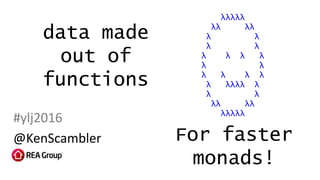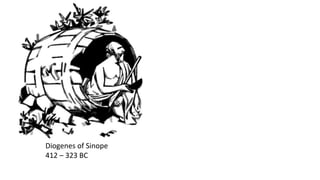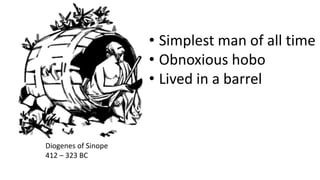Diese Präsentation wurde erfolgreich gemeldet.

# Data made out of functions

Anzeige
Anzeige
Anzeige
Anzeige
Anzeige
Anzeige
Anzeige
Anzeige
Anzeige
Anzeige
Anzeige×

## Hier ansehen

1 von 101 Anzeige

# Data made out of functions

Some examples and motivation for creating data structures from nothing but functions - Church Encoding! There's particular detail on how it can make free monads more efficient.

Some examples and motivation for creating data structures from nothing but functions - Church Encoding! There's particular detail on how it can make free monads more efficient.

Anzeige
Anzeige

### Data made out of functions

1. 1. data made out of functions #ylj2016 @KenScambler λλλλλ λλ λλ λ λ λ λ λ λ λ λ λ λ λ λ λ λ λ λλλλ λ λ λ λλ λλ λλλλλ For faster monads!
2. 2. Diogenes of Sinope 412 – 323 BC
3. 3. Diogenes of Sinope 412 – 323 BC • Simplest man of all time • Obnoxious hobo • Lived in a barrel
4. 4. I’ve been using this bowl like a sucker!
5. 5. Um…. what
6. 6. "abcd" IF x THEN y ELSE z WHILE cond {…} [a, b, c, d] BOOL INT STRUCT { fields… } λa -> b
7. 7. IF x THEN y ELSE z WHILE cond {…} [a, b, c, d] BOOL INT STRUCT { fields… } λa -> b Strings are pretty much arrays
8. 8. IF x THEN y ELSE z [a, b, c, d] BOOL INT STRUCT { fields… } λa -> b Recursion can do loops
9. 9. IF x THEN y ELSE z [a,b,c,d] BOOL INT STRUCT { fields… } λa -> b Recursive data structures can do lists
10. 10. IF x THEN y ELSE z [a,b,c,d] INT STRUCT { fields… } λa -> b Ints can do bools
11. 11. IF x THEN y ELSE z [a,b,c,d] INT STRUCT { fields… } λa -> b
12. 12. [a,b,c,d] STRUCT λa -> b
13. 13. Alonzo Church 1903 - 1995 λa -> b Lambda calculus
14. 14. λa -> b Alonzo Church 1903 - 1995 Lambda calculus
15. 15. We can make any data structure out of functions! Church encoding
16. 16. Booleans Bool
17. 17. Church booleans resultBool
18. 18. Church booleans resultBool If we define everything you can do with a structure, isn’t that the same as defining the structure itself?
19. 19. TRUE FALSE or
20. 20. TRUE FALSE or result “What we do if it’s true”
21. 21. “What we do if it’s false” TRUE FALSE or result
22. 22. TRUE FALSE or result result result
23. 23. () () result result result
24. 24. result result result
25. 25. r r r The Church encoding of a boolean is:
26. 26. type CBool = forall r. r -> r -> r cTrue :: CBool cTrue x y = x cFalse :: CBool cFalse x y = y cNot :: CBool -> CBool cNot cb = cb cFalse cTrue cAnd :: CBool -> CBool -> CBool cAnd cb1 cb2 = cb1 cb2 cFalse cOr :: CBool -> CBool -> CBool cOr cb1 cb2 = cb1 cTrue cb2
27. 27. Natural numbers 0 1 2 3 4 …
28. 28. Natural numbers 0 0 +1 0 +1 +1 0 +1 +1 +1 0 +1 +1 +1 +1 …
29. 29. Natural numbers 0 0 +1 0 +1 +1 0 +1 +1 +1 0 +1 +1 +1 +1 … Giuseppe Peano 1858 - 1932 Natural numbers form a data structure!
30. 30. Zero Succ(Nat) or Nat = Natural Peano numbers Giuseppe Peano 1858 - 1932
31. 31. or Nat = Now lets turn it into functions! Zero Succ(Nat)
32. 32. Zero Succ(Nat) or result “If it’s a successor”
33. 33. or “If it’s zero” resultZero Succ(Nat)
34. 34. result result resultor Zero Succ(Nat)
35. 35. Nat () result result result
36. 36. Nat result result result
37. 37. Nat result result result() Nat () Nat () Nat () Nat
38. 38. result result result result
39. 39. (r r) r The Church encoding of natural numbers is: r
40. 40. type CNat = forall r. (r -> r) -> r -> r c0, c1, c2, c3, c4 :: CNat c0 f z = z c1 f z = f z c2 f z = f (f z) c3 f z = f (f (f z)) c4 f z = f (f (f (f z))) cSucc :: CNat -> CNat cSucc cn f = f . cn f cPlus :: CNat -> CNat -> CNat cPlus cn1 cn2 f = cn1 f . cn2 f cMult :: CNat -> CNat -> CNat cMult cn1 cn2 = cn1 . cn2
41. 41. type CNat = forall r. (r -> r) -> r -> r c0, c1, c2, c3, c4 :: CNat c0 f = id c1 f = f c2 f = f . f c3 f = f . f . f c4 f = f . f . f . f cSucc :: CNat -> CNat cSucc cn f = f . cn f cPlus :: CNat -> CNat -> CNat cPlus cn1 cn2 f = cn1 f . cn2 f cMult :: CNat -> CNat -> CNat cMult cn1 cn2 = cn1 . cn2
42. 42. Performance Native ints Peano numbers Church numbers addition print O(n) O(n2) multiplication O(n) O(n) O(1) O(1)
43. 43. Performance Native ints Peano numbers Church numbers addition print O(n) O(n2) multiplication O(n) O(n) O(1) O(1)
44. 44. Church encoding cheat sheet A | B (A, B) Singleton Recursion (a r) (b r) r (a r)b r r r r A a r
45. 45. Nil Cons(a, List a) or List a = Cons lists
46. 46. Nil Cons(a, List a) or result result result
47. 47. (a, List a) result result result ()
48. 48. (a, ) result result result result
49. 49. a result result result result
50. 50. r r The Church encoding of lists is: r(a ) r
51. 51. r r The Church encoding of lists is: r(a ) r AKA: foldr
52. 52. Functors a
53. 53. Functors f a a
54. 54. Functors f (f a) They compose! f a a
55. 55. Functors f (f (f a)) What if we make a “Church numeral” out of them? f (f a) f a a
56. 56. Free monads f (f (f (f a))) f (f (f a)) f (f a) f a a
57. 57. Free monad >>= a
58. 58. Free monad >>= a fmap
59. 59. Free monad >>= f a
60. 60. Free monad >>= f a fmap
61. 61. Free monad >>= f a fmap
62. 62. Free monad >>= f (f a)
63. 63. Free monad >>= f (f a) fmap
64. 64. Free monad >>= f (f a) fmap
65. 65. Free monad >>= f (f a) fmap
66. 66. Free monad >>= f (f (f a))
67. 67. Free monad >>= f (f (f a)) fmap
68. 68. Free monad >>= f (f (f a)) fmap
69. 69. Free monad >>= f (f (f a)) fmap
70. 70. Free monad >>= f (f (f a)) fmap
71. 71. λn  [n+1, n*2] 3
72. 72. λn  [n+1, n*2] 4 6
73. 73. λn  [n+1, n*2] 4 6 fmap
74. 74. λn  [n+1, n*2] 5 8 7 12
75. 75. λn  [n+1, n*2] 5 8 7 12 fmap
76. 76. λn  [n+1, n*2] 5 8 7 12 fmap
77. 77. λn  [n+1, n*2] 6 10 9 16 8 14 13 24
78. 78. λn  Wrap [Pure (n+1), Pure (n*2)] 3
79. 79. λn  Wrap [Pure (n+1), Pure (n*2)] >>=3
80. 80. 4 6 λn  Wrap [Pure (n+1), Pure (n*2)]
81. 81. 4 6 λn  Wrap [Pure (n+1), Pure (n*2)] >>=
82. 82. 4 6 λn  Wrap [Pure (n+1), Pure (n*2)] fmap
83. 83. 4 6 λn  Wrap [Pure (n+1), Pure (n*2)] >>=
84. 84. λn  Wrap [Pure (n+1), Pure (n*2)] 5 8 7 12
85. 85. λn  Wrap [Pure (n+1), Pure (n*2)] 5 8 7 12 >>=
86. 86. λn  Wrap [Pure (n+1), Pure (n*2)] 5 8 7 12 fmap
87. 87. λn  Wrap [Pure (n+1), Pure (n*2)] 5 8 7 12 >>=
88. 88. λn  Wrap [Pure (n+1), Pure (n*2)] 5 8 7 12 fmap
89. 89. λn  Wrap [Pure (n+1), Pure (n*2)] >>=5 8 7 12
90. 90. λn  Wrap [Pure (n+1), Pure (n*2)] 6 10 9 16 8 14 13 24
91. 91. Pure a Wrap f (Free f a) or Free a = Free monads
92. 92. Pure a Wrap f (Free f a) or result result result
93. 93. f (Free f a) result result result a
94. 94. f result result result a result
95. 95. r r The Church encoding of free monads is: (f ) rr(a )
96. 96. r r(f ) rr(a ) >>= CFree f b Bind is constant time!
97. 97. λa -> b
98. 98. λa -> b ∴
99. 99. λa -> b ∴